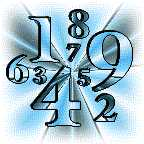## The WizardThe Wizard requests that you do the following:
• Select any integer greater than 1 but less than 1000.
• Multiply that integer by 9 to get your Multiplied Number.
• Your Multiplied Number has either 2,3 or 4 digits. Add these digits together. If the result is a single digit, call that result your Reference Number . If the result of the digit summation yields two digits, again add these together to get your Reference Number .
• Subtract 5 from your Reference Number to get your Base Number . If this result is less than zero, first add 8 to get your Base Number .
• Make the identification 1 = A, 2 = B, 3 = C, 4 = D, 5 = E, etc.., to obtain your Base Letter .
• Think of a country that starts with your Base Letter , and of an animal that starts with the last letter of that country. If you can name several animals, select the largest in size.

Now that you've selected a country and an animal, Visit The Wizard